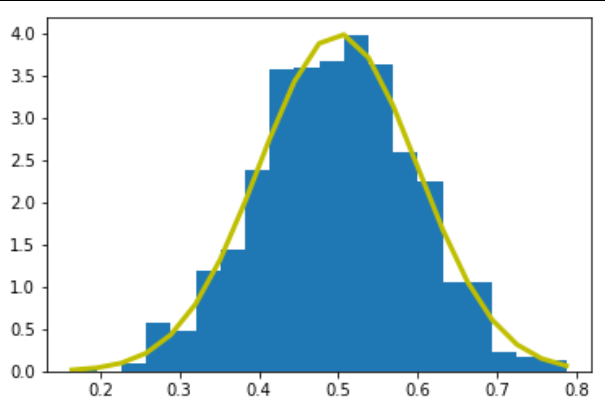# Python - Normal Distribution

The normal distribution is a form presenting data by arranging the probability distribution of each value in the data.Most values remain around the mean value making the arrangement symmetric.

We use various functions in numpy library to mathematically calculate the values for a normal distribution. Histograms are created over which we plot the probability distribution curve.

```import matplotlib.pyplot as plt
import numpy as np

mu, sigma = 0.5, 0.1
s = np.random.normal(mu, sigma, 1000)

# Create the bins and histogram
count, bins, ignored = plt.hist(s, 20, normed=True)

# Plot the distribution curve
plt.plot(bins, 1/(sigma * np.sqrt(2 * np.pi)) *
np.exp( - (bins - mu)**2 / (2 * sigma**2) ),       linewidth=3, color='y')
plt.show()
```

Its output is as follows −